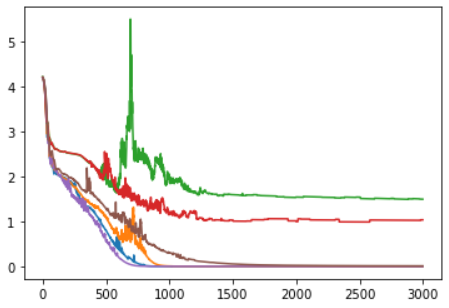# Teacher forcing with model.eval()

I have a seq2seq LSTM model with 100% teacher forcing. The loss decreases nicely as time progresses. However, when I place my model into model.eval() mode & without teacher forcing, the eval loss diverges (increases) away from the training loss (decreases) as time progresses.

I have tried using learning_rate schedulers during training e.g. exponential probability, or 0.5 probability of teacher forcing at a given time step, this resulted in no change to the eval-train trend.

I then tried the following:

• train data == eval data, results: same trend
• remove batch shuffling, results : same trend
• vary batch size, results: same trend
• vary data set size, results: same trend

Note:
I do not have batch normalization in my model.
Putting teacher forcing in my eval function gives same eval loss as training loss.

Is there anything that could explain such results aside from coding errors (I have unit tests at each stage).

Thanks.

Based on your description it seems that teacher forcing gives your model a strong signal, so that the “underlying” distribution wasn’t learned from previously predicted samples.
How does the training loss look when you don’t use teacher forcing or with a low probability?

Terrible, converges to very high loss (start: 4, converges:2). I have also tried to force the model to over-fit by increasing the layers and hidden units. Yet without teacher forcing the model doesn’t improve. I’m at a bit of a loss, the only other thing I can consider is:

Change internals:
Deviate from the tutorial, instead of feeding only the final GRU/LSTM h_state to the decoder. I could try feeding in each encoder layer h_state to the corresponding layers in the decoder.